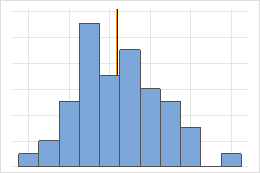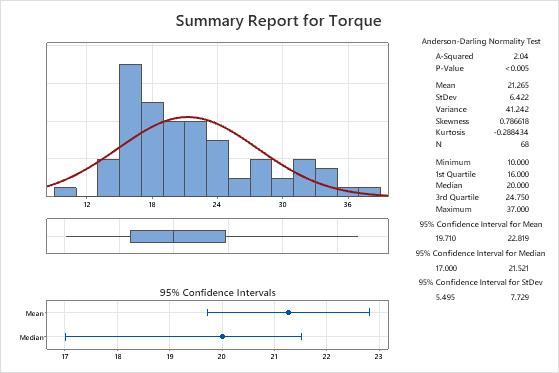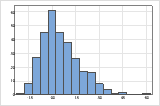# Interpret the key results for Graphical Summary

Complete the following steps to interpret graphical summary. Key output includes the p-value, the mean, the median, the confidence interval, and several graphs.

## Step 1: Describe the center of your data

Use the mean to describe the sample with a single value that represents the center of the data. Many statistical analyses use the mean as a standard measure of the center of the distribution of the data.

The median is another measure of the center of the distribution of the data. The median is usually less influenced by outliers than the mean. Half the data values are greater than the median value, and half the data values are less than the median value.

The median and the mean both measure central tendency. But unusual values, called outliers, can affect the median less than they affect the mean. If your data are symmetric, the mean and median are similar.For the symmetric distribution, the mean (blue line) and median (orange line) are so similar that you can't easily see both lines. But the non-symmetric distribution is skewed to the right.Key Results: Mean and MedianIn these results, the mean torque that is required to remove a toothpaste cap is 21.265, and the median torque is 20. The data appear to be skewed to the right, which explains why the mean is greater than the median.

## Step 2: Determine a confidence interval for the mean, median, and standard deviation

The confidence interval provides a range of likely values for the population parameter. For example, a 95% confidence level indicates that if you take 100 random samples from the population, you could expect approximately 95 of the samples to produce intervals that contain the population parameter.Key Results: Confidence Interval for Mean, Confidence Interval for Median, Confidence Interval for StDev In these results, the confidence intervals indicate that you can be 95% confident of the following: The population mean for the torque measurements is between 19.710 and 22.819. The population median for the torque measurements is between 17 and 21.521. The population standard deviation for the torque measurements is between 5.495 and 7.729.

## Step 3: Assess the shape and spread of your data distribution

Use the histogram and boxplot to assess the shape and spread of the data, and to identify any potential outliers.

When data are skewed, the majority of the data are located on the high or low side of the graph. Often, skewness is easiest to detect with a histogram or boxplot.The histogram with right-skewed data shows wait times. Most of the wait times are relatively short, and only a few wait times are long. The histogram with left-skewed data shows failure time data. A few items fail immediately, and many more items fail later.

### Identify outliers

Outliers, which are data values that are far away from other data values, can strongly affect the results of your analysis. Often, outliers are easiest to identify on a boxplot.